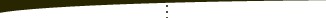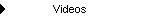About   Products   Support   Videos   Purchase   ContactThe Breakout Bulletin The following article was originally published in the July 2003 issue of The Breakout Bulletin.   Fixed Ratio Position Sizing In my previous discussions of position sizing, I've always assumed that the number of contracts was determined based on the risk of the trade. For example, you might decide to risk 3% of your trading equity on the next trade. If the trade has a potential loss of \$500 per contract, and your account equity is \$50,000, you would take 3% of your equity (0.03 * \$50,000 = \$1,500) and divide the result by the trade risk of \$500. The result is \$1,500/\$500 = 3 contracts. This is known as fixed fractional position sizing and is widely used in futures trading.    Basing position sizing on risk makes intuitive sense in that we know the greater the risk, the greater the reward. We expect that if we risk more of our equity on each trade, we will earn a higher return. This is the case with fixed fractional trading, provided we don't risk more than the so-called "optimal f" (see reference 1) and presuming our trading method is inherently profitable. Fixed fractional trading also helps us relate the risk of individual trades to the drawdown risk. By drawdown risk, I mean the largest percentage decline in equity from the most recent equity peak. Most traders have a limit to how much drawdown they can tolerate; e.g., 30%. By using a method like Monte Carlo simulation, which I discussed last month, it's possible to relate the trade risk, as represented by the fixed fraction, to the drawdown risk.   However, fixed fractional position sizing is not the only method of position sizing available. I often get questions about fixed ratio position sizing, so this month, I'll discuss the concept of fixed ratio position sizing and compare it to the fixed fractional method. In his book "The Trading Game" (reference 2), Ryan Jones introduced the fixed ratio method, which he developed to address some of the limitations he felt existed in fixed fractional position sizing.   The key concept of the fixed ratio method is the delta. The delta is the profit per contract needed to increase the number of contracts by one. For example, starting with one contract and with a delta of \$5,000, you need a profit of \$5,000 to increase the number of contracts to two. With two contracts, you need a profit per contract of \$5,000 or \$10,000 total from the two contracts to increase the number of contracts to three. With three contracts, you need a profit of \$15,000 to increase the number of contracts to four. With four contracts, you need to profit of \$20,000 to increase the number of contracts to five, and so on.   Based on the relationships presented by Jones, it's possible to derive the following equation for the number of contracts in fixed ratio position sizing:    N = 0.5  * [1 + (1 + 8 * Profit/delta)^0.5]   where Profit  = total closed trade profit in dollars, delta = profit/contract to increase by one contract, and "^0.5" means that the expression in parentheses is raised to the power of 0.5.   It's interesting to compare this equation to the corresponding equation for fixed fractional trading:       N = ff * Equity/| trade risk |   where "trade risk" is the possible loss in dollars for the trade, and the vertical bars (|) represent absolute value. Notice that the relationship between the number of contracts and the profit is linear with fixed fractional trading. As the profits accrue, the number of contracts increases linearly. The rate of change of N with respect to account equity is constant with the fixed fractional method; e.g., a \$10,000 increase in profits results in the same increase in the number of contracts regardless of whether that profit occurred with a \$15,000 account or a \$150,000 account.   With fixed ratio trading, on the other hand, as you accrue more profits, the number of contracts increases more slowly. A \$10,000 profit with a \$20,000 account will increase the number of contracts more than if a \$10,000 profit is made on a \$200,000 account. For small account sizes, you'll increase the number of contracts more quickly with fixed ratio position sizing. However, when the account equity becomes larger, the number of contracts will increase more slowly than with fixed fractional position sizing. This is why fixed ratio position sizing is sometimes preferred for small accounts.   Because the fixed ratio method depends on account size, how it performs compared to the fixed fractional method over a series of trades depends on where the drawdowns occur. If the biggest drawdown occurs late in the sequence of trades, the fixed ratio method will do well because the fastest increase in the number of contracts will have occurred during the most profitable period. On the other hand, if the biggest runup in equity occurs late in the sequence of trades, the fixed fractional method will do better because it will increase the number of contracts more quickly at that point, whereas the rate of increase in the number of contracts with the fixed ratio method will have already slowed.   As an example, consider the following equity curve from a real sequence of trades. I adjusted the delta for the fixed ratio method and the fixed fraction for the fixed fractional method so that the worst-case percentage drawdown was the same in each case. Fig. 1 shows the equity curves for both methods when the trades occur in their historical sequence. The fixed ratio method clearly delivers superior performance. The net profit is much higher for the same maximum drawdown. Note that the primary run up in equity occurred early in the sequence of trades. The fixed ratio method was more aggressive early on when it mattered the most.Figure 1. Equity curve for historical sequence of trades using fixed fractional ("Fix Fract") and fixed ratio ("Fix Ratio") position sizing. The maximum peak-to-valley drawdown is the same (in percentage terms) in each case.   However, if we randomize the trade sequence, as in Fig. 2, the opposite result is possible. These are the same trades as in Fig. 1, just in a different order. Again, the parameters for the two methods have been adjusted to produce the same maximum peak-to-valley percentage drawdown. In this case, the fixed fractional method generates a much higher return for the same drawdown. In this sequence of trades, the run up in equity occurred late in the sequence. As a result, the fixed fractional method was more aggressive than the fixed ratio method in increasing the number of contracts late in the sequence when it counted most.Figure 2. Equity curve for randomized sequence of the same trades as in Fig. 1 using fixed fractional ("Fix Fract") and fixed ratio ("Fix Ratio") position sizing. The maximum peak-to-valley drawdown is the same (in percentage terms) in each case.   For any sequence of trades, one method will be better than the other. However, even if we have a good idea of the distribution of our trades, the sequence is always unknown. As I discussed in last month's newsletter, one way to deal with the consequences of not knowing the sequence of a series of trades is to use the Monte Carlo method. With the Monte Carlo method, we can perform an analysis over many different, randomly chosen trade sequences and evaluate the results in terms of statistics. In effect, this is a way to convert the uncertainty of the trade sequence into a quantified (if probabilistic) result. This method might be able to tell us whether the fixed fractional or fixed ratio method is better for a given series of trades.   We can take this analysis one step further by reconsidering the equation presented above for the fixed ratio method. Notice the 0.5 exponent in the equation for the number of contracts, N, in the fixed ratio method. Consider what we would get if the 0.5 was replaced with 0. In that case, we get N = 1. In other words, an exponent of zero represents fixed contract trading with one contract per trade. What if the exponent has the value 1? In this case, we find that the number of contracts, N, is proportional to the profit. This is the basis of fixed fractional trading. In other words, an exponent of 1 represents fixed fractional position sizing.   There's nothing preventing us from choosing other exponent values as well. With this in mind, we can write a more generalized form of the position sizing equation as:   N = 0.5  * [1 + (1 + 8 * Profit/delta)^m]   where the exponent m can vary from 0 to any positive number we like. With m = 0, we get fixed contract trading. With m = 1, we have the equivalent of fixed fractional trading. m = 0.5 gives us fixed ratio trading.   Any value of m less than 1 (e.g., 0.5 or 0.10) will increase the number of contracts more slowly for larger account equity values. Values of m larger than 1 will increase the number of contracts more quickly as the account equity increases. At m = 1 (i.e., fixed fractional), the rate of change in the number of contracts is independent of account size.   We might expect that for any sequence of trades, there's an "optimal" value of m. By optimal, I mean there's one value of m that produces the greatest return for a given maximum drawdown. As noted above, since we don't know the sequence of trades to expect in the future, calculating this optimal m for a historical sequence of trades is probably a pointless exercise. However, it might be interesting to use the Monte Carlo method to see what this optimal m would be based on the statistical results of the Monte Carlo simulation. I've yet to do that analysis, however.   That's all for now. Good luck with your trading.   References: Ralph Vince, Portfolio Management Formulas, John Wiley & Sons, Inc., New York, 1990. Ryan Jones, The Trading Game, John Wiley & Sons, Inc., New York, 1999.   Mike Bryant Breakout FuturesCopyright © 2001-2013 Breakout Futures Copyright © 2013-2017 Adaptrade Software